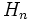# Lower central series is fastest descending central series

## Statement

Suppose$G$ is a Nilpotent group (?) with a Central series (?):$G = H_1 \ge H_2 \ge \dots H_n = \{ e \}$

Then, if we consider the Lower central series (?) of$G$:$G_1 = G, G_{i+1} = [G_i,G]$

Then, for every$i$, we have:$H_i \ge G_i$

In particular, if$G$ has Nilpotence class (?)$c$, then:$n \ge c + 1$

## Facts used

We use the following fact:

• If$H_1 \le K_1 \le G$ and$H_2 \le K_2 \le G$, then$[H_1,H_2] \le [K_1,K_2]$.

## Proof

Given':$G$ is a nilpotent group with a central series:$G = H_1 \ge H_2 \ge \dots H_n = \{ e \}$

Consider the lower central series of$G$:$G_1 = G, G_{i+1} = [G_i,G]$

To prove: For every$i$, we have:$H_i \ge G_i$

In particular, if$G$ has nilpotence class$c$, then:$n \ge c + 1$

Proof: We prove this by induction on$i$.

Base case for induction: For$i = 1$,$H_1 = G_1 = G$ so it's true.

Induction step: Suppose$H_i \ge G_i$. Then by the definition of the lower central series:$[G,G_i] = G_{i+1}$

Because$H_i \ge G_i$, we have, by the fact mentioned above:$[G,H_i] \ge [G,G_i]$

Finally, by the definition of central series, we have:$H_{i+1} \ge [G,H_i]$

Combining these facts, we see that:$H_{i+1} \ge G_{i+1}$

Thus, if$H_n$ is trivial,$G_n$ must be trivial. Since the smallest$n$ for which$G_n$ is trivial is$c + 1$, we have$n \ge c + 1$.